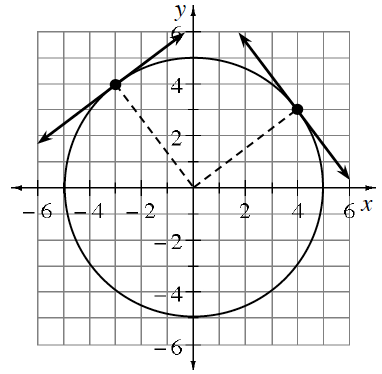### Home > PC3 > Chapter 11 > Lesson 11.1.4 > Problem11-72

11-72.

Two lines are drawn tangent to the circle $x^{2} + y^{2} = 25$, one at the point $\left(4, 3\right)$ and the other at the point $\left(−3, 4\right)$. What is the point of intersection for the two tangent lines?

Draw a diagram. Recall that a line tangent to a circle is perpendicular to the radius at that point.